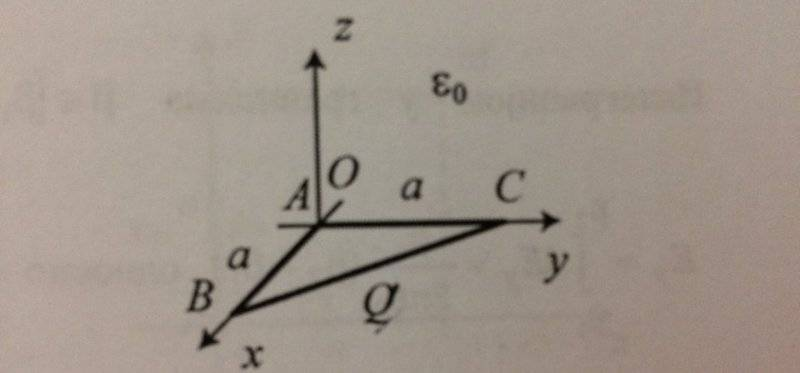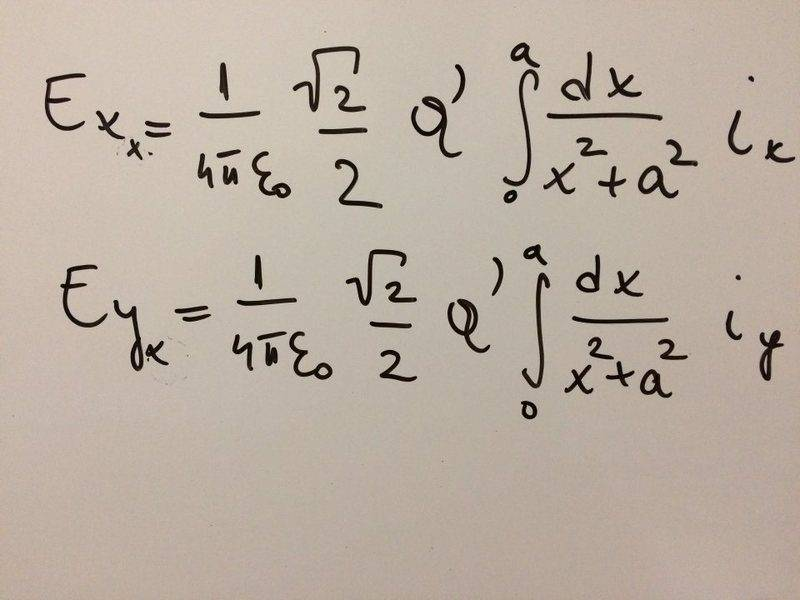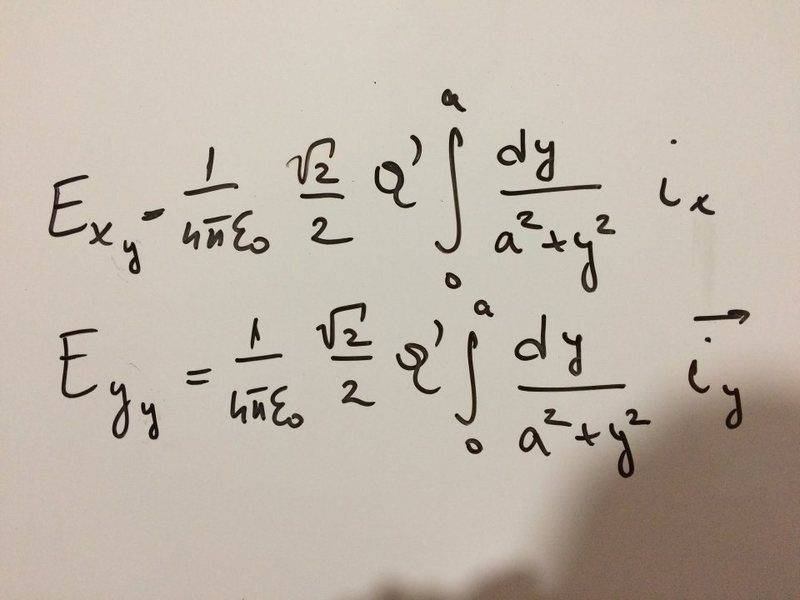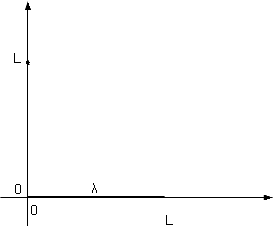# Calculate the electric field using superposition

• diredragon

## Homework Statement

Find the expression for the electric field at point M(a,a,0) if the linear charge density is known ( ##Q'## )## Homework Equations

3. The Attempt at a Solution [/B]
I tried something like this and would like your feedback on it. I separated the triangle into three parts. First i consider only the side on the x-axis and calculate that field.
##E_x=E_{Xx}i_x + E_{Yx}i_y## I hope the notation is clear because i use ##E_x## as the total electric field of a x segment of the triangle and the ##E_{Xx}, E_{Yx}## as the vector compositions of the mention vector. Since its a square they are each ##E_x\frac{\sqrt{2}}{2}##. The same i wrote for the ##E_y## segment as that segment only adds on the vectors on the x segment but are integrated by ##dy## (see the picture). Does this make sense and is it worthwhile to continue adding the third segment, which will have to be integrated by ##dy## and ##dx##? I think i could do tCould you help me with this, it has been struggling with me for a while now xD..Where is M? You wrote (a, a, 0), but as it is not shown in the diagram I'd like you to confirm that.
I do not understand how you get your integrands. What is the magnitude of the field at M due to an element dx at distance x from O on the X axis? What are the X and Y components of that?

The M is not shown but i confirm that that is the point where electric field is to be calculated. I can explain what i did like this:
Consider only the x-axis and the point. The Electric field do the element ##dx## is ##dE=\frac{Q'dx}{4πεr^2}##, ##r^2=a^2+x^2## and ##r## is the distance from some point on x-axis to point M. The total electric field is the integral of all elements ##dE## from ##x=0## to ##x=a##. The components of this vector are acting in x and y directions and are both equal in magnitude ##dE_x=dE_y=dEcos45=dE\frac{\sqrt{2}}{2}##

The total electric field is the integral of all elements dE from x=0 to x=a.
Yes, but that is integrating a vector. The fields from the dx elements do not all point in the same direction. You need to break it into components before integrating.

Yes, but that is integrating a vector. The fields from the dx elements do not all point in the same direction. You need to break it into components before integrating.
I thought that was what i did in the pictures i posted.. broke the electric field vector from the x segment into its components, the x and y of that vector? They are the same in magnitude as i wrote but point in different directions as indicated by ix and iy unit vectors..right?

I thought that was what i did in the pictures i posted.. broke the electric field vector from the x segment into its components, the x and y of that vector? They are the same in magnitude as i wrote but point in different directions as indicated by ix and iy unit vectors..right?
No, you seem to have integrated wrt x before breaking it into components. That is not valid because you integrated a vector as though the direction was constant. The field due to each little dx points in a different direction.
The magnitude of the field at M from an element dx at distance x from B is ##\frac{kQ'dx}{a^2+x^2}##. What direction is it in? What are its X and Y components?

No, you seem to have integrated wrt x before breaking it into components. That is not valid because you integrated a vector as though the direction was constant. The field due to each little dx points in a different direction.
The magnitude of the field at M from an element dx at distance x from B is ##\frac{kQ'dx}{a^2+x^2}##. What direction is it in? What are its X and Y components?
##\frac{kQ'dxa}{(a^2+x^2)r}## i put the angle to be constant where it isnt. Now its ##a/r## right?

##\frac{kQ'dxa}{(a^2+x^2)r}## i put the angle to be constant where it isnt. Now its ##a/r## right?
Where r is ##\sqrt(a^2+x^2)##, that's one component, yes.

Where r is ##\sqrt(a^2+x^2)##, that's one component, yes.
The second component would then be the same but with ##x/r## factor. The same is true for the y segment components but they are integrated along ##dy## right?

The second component would then be the same but with ##x/r## factor. The same is true for the y segment components but they are integrated along ##dy## right?
Yes.

I can't seem to get the third segment right. I am integrating along ##dL## that is the length of that side but ##dL^2=dx^2+dy^2## so i have to separate the integral into two parts, the x and y and then calculate the components?

I can't seem to get the third segment right. I am integrating along ##dL## that is the length of that side but ##dL^2=dx^2+dy^2## so i have to separate the integral into two parts, the x and y and then calculate the components?
The easiest way to do that one is through a change of axes.

Yes.

The easiest way to do that one is through a change of axes.
Can i see that third segment as some z axis and the point M as being distance ##a\sqrt{2}/2## from the middle? The electric field would disappear along the z axis but would exist on the axis normal to that z? Then i would see hows that z axis connected to the other two?

third segment as some z axis and the point M as being distance ##\sqrt{2}/2 ## from the middle?
The distance from M to midpoint of BC is not ##\sqrt{2}/2 ##
The electric field would disappear along the z axis but would exist on the axis normal to that z?
Yes. Figuring out how to put the field back into components along the original axes might be the hard part.

## Homework Statement

Find the expression for the electric field at point M(a,a,0) if the linear charge density is known ( ##Q'## )
View attachment 107346

## Homework Equations

3. The Attempt at a Solution [/B]
I tried something like this and would like your feedback on it. I separated the triangle into three parts. First i consider only the side on the x-axis and calculate that field.
##E_x=E_{Xx}i_x + E_{Yx}i_y## I hope the notation is clear because i use ##E_x## as the total electric field of a x segment of the triangle and the ##E_{Xx}, E_{Yx}## as the vector compositions of the mention vector. Since its a square they are each ##E_x\frac{\sqrt{2}}{2}##. The same i wrote for the ##E_y## segment as that segment only adds on the vectors on the x segment but are integrated by ##dy## (see the picture). Does this make sense and is it worthwhile to continue adding the third segment, which will have to be integrated by ##dy## and ##dx##? I think i could do it. Could you help me with this, it has been struggling with me for a while now xD..
View attachment 107347
View attachment 107348

Judging by the title of this thread, I suspect that the idea here is to actually use superposition to solve this problem.

Break the linear charge distribution into 4 segments. All 4 segments have the same orientation relative to the observation point, as well as all subtend the same angle, namely π/4, (45°) .

Consider a linear charge of uniform linear density, λ, and length, L, lying along the x-axis, with one end at the origin and the other x = L . Determine the electric field at a point on the y-axis a distance of L from the origin. There will be a component in the y direction and a component in the x direction.

Use this result along with superposition to solve your problem.

I suspect that the idea here is to actually use superposition
I thought that was how the thread was progressing.

I thought that was how the thread was progressing.
I did not detect that.

The distance from M to midpoint of BC is not ##\sqrt{2}/2 ##

Yes. Figuring out how to put the field back into components along the original axes might be the hard part.
I ment ##\frac{a\sqrt{2}}{2}## to be the distance and i think it would be easy to transit from z to original. If i place the line along z axis and imagine that the normal to z is some G axis i get the electric field only aling that G couse the Z component cancels out. Imagining that it should be that ##(E_g)^2=(E_x)^2 + (E_y)^2## (components of the z line segment ) where the components are the same because of the fact that the point is at middle from the line. Then they are each in positive respective original axis direction and equal to the product of the magnitude of ##E_g##, ##cos(45)## and respective unit vector..##i_x, i_y##. This seems correct, what do you think?

The distance from M to midpoint of BC is not ##\sqrt{2}/2 ##
Sorry, that was a mistake. For some reason I keep thinking M is at (0,0,a).

I ment ##\frac{a\sqrt{2}}{2}## to be the distance and i think it would be easy to transit from z to original. If i place the line along z axis and imagine that the normal to z is some G axis i get the electric field only aling that G couse the Z component cancels out. Imagining that it should be that ##(E_g)^2=(E_x)^2 + (E_y)^2## (components of the z line segment ) where the components are the same because of the fact that the point is at middle from the line. Then they are each in positive respective original axis direction and equal to the product of the magnitude of ##E_g##, ##cos(45)## and respective unit vector..##i_x, i_y##. This seems correct, what do you think?
Not sure I followed all that. Just post your working, that will be clearer.

Not sure I followed all that. Just post your working, that will be clearer.
I used a lot of symbols so ask if something need clearing up. Basically this would calculate the resulatant electric field generated by the third line in the coordinates that were made up. If this is correct all i need to do now is transit the third vector to the original coordinates and add up its components where they need to be added up.

#### Attachments

As I previously posted:
Judging by the title of this thread, I suspect that the idea here is to actually use superposition to solve this problem.

Break the linear charge distribution into 4 segments. All 4 segments have the same orientation relative to the observation point, as well as all subtend the same angle, namely π/4, (45°) .

Consider a linear charge of uniform linear density, λ, and length, L, lying along the x-axis, with one end at the origin and the other x = L . Determine the electric field at a point on the y-axis a distance of L from the origin. There will be a component in the y direction and a component in the x direction.

Use this result along with superposition to solve your problem.
Maybe the following sketch will help.Find the electric field at y = L on the y-axis.

Find each component.

I used a lot of symbols so ask if something need clearing up. Basically this would calculate the resulatant electric field generated by the third line in the coordinates that were made up. If this is correct all i need to do now is transit the third vector to the original coordinates and add up its components where they need to be added up.
Again, you appear to have integrated the magnitude of the field (##\int \frac{dz}{c^2+z^2}##) instead of its components. All your integrals should be of the form ##\int\frac{cdz}{(c^2+z^2)^\frac 32}## or ##\int\frac{zdz}{(c^2+z^2)^\frac 32}##, according to whether the component is normal to the wire segment or parallel to it.

I used a lot of symbols so ask if something need clearing up. Basically this would calculate the resulatant electric field generated by the third line in the coordinates that were made up. If this is correct all i need to do now is transit the third vector to the original coordinates and add up its components where they need to be added up.

Again, you appear to have integrated the magnitude of the field (##\int \frac{dz}{c^2+z^2}##) instead of its components. All your integrals should be of the form ##\int\frac{cdz}{(c^2+z^2)^\frac 32}## or ##\int\frac{zdz}{(c^2+z^2)^\frac 32}##, according to whether the component is normal to the wire segment or parallel to it.
I posted it above but missed to put in in the formula, the term missing in the integral is ##\frac{a\sqrt{2}}{2r}## and this should be the right form?

The second component would then be the same but with ##x/r## factor. The same is true for the y segment components but they are integrated along ##dy## right?
My earlier point regarding superposition was that you only need to do the one pair of integrations needed for getting the E field due to what you call the x segment.

You can use superposition and scaling to complete the solution using that result.

What are your results for each of the following?
##\displaystyle
\int_0^a \frac{a\,dx}{(a^2+x^2)^\frac 32} ##

##\displaystyle \int_0^a \frac{x\,dx}{(a^2+x^2)^\frac 32}##

Last edited:
My earlier point regarding superposition was that you only need to do the one pair of integrations needed for getting the E field due to what you call the x segment.

You can use superposition and scaling to complete the solution using that result.

What are your results for each of the following?
##\displaystyle
\int_0^a \frac{a\,dx}{(a^2+x^2)^\frac 32} ##

##\displaystyle \int_0^a \frac{x\,dx}{(a^2+x^2)^\frac 32}##
What segment are we talking about? The first line along the x-axis?

What segment are we talking about? The first line along the x-axis?
It's set up as the x-segment isn't it?

But it really doesn't matter. What do you get for each of those?